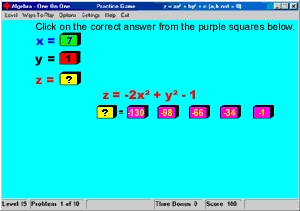# Algebra - One On One Description

Algebra One on One is an educational game for those wanting a fun way to learn and practice Algebra. This program covers 21 functions which includes maximums, minimums, absolute values, averages, x/y, ax + b, axy + b, ax + by + c, squares, cubes, and so on. It has a practice and a game area.It has a great help system that makes it easy for the beginner to do and understand algebra. It also has a"Einstein"level that even algebra experts will find fun and challenging. You can choose from a ten problem, a time trial, or a two-player game. High scores are saved and you are given a rank according to your score. The ranks are Novice, Learner, Veteran, Calculator, Math Pro, Math Whiz, Math Genius, and Einstein.The practice menu lets you practice each function individually. The game menu lets you choose one function, two functions, and so on up to 21 functions. You can choose from calculate value (1 x and 1y value and the equation to solve), choose formula (you figure out the equation using the given x, y, and z values), or figure formula and calculate (you figure out the equation and solve for the missing z value).If you get stuck trying to figure out what the function (equation) is a hint will be displayed. If you choose the wrong answer it will help you figure out the right one. The calculate option combined with the practice game enables students to practice solving the problems in the area they are having trouble with.

Category: Education And Reference > Math

Size: 1MB

OS: Win 95/98/NT/2000# MITCalc - Profiles Calculation

The calculation solves area characteristics of profiles and mass characteristics of solids created by extrusion or
rotation of the profile. Application is developed in MS Excel, is multi-language and supports Imperial and Metric
units.

# Multimedia Calculator

MC is an ideal alternative calculator.

# 3D Geometrical Objects

The program can represent the following objects in 3D space: regular prism (3 to 10 sides); general three-sided prism; special four-sided prism (adjustable inclination of sides); regular pyramid (3 to 10 sides). Interactive 3D animation.